#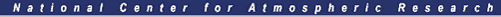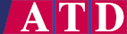Sounding Data Analysis

### Background Information

In addition to the sounding data processing described previously, some rudimentary analysis has been performed on the sounding data. This effort was separate from the processing described elsewhere and utilizes an interactive data analysis package known as "SUDS" which has been created and maintained by the NCAR Research Data Program.

"SUDS" reads, plots, edits, and analyzes sounding data. The "SUDS" program was run on each sounding (the final "Interpolated Data" file) to generate several parameters. No data editing was done to avoid possible confusion. If parameters obtained in the analysis are bad due to bad data, they are left that way.

The parameters produced from the "SUDS" analysis are: surface potential temperature (50 mb average); surface virtual potential temperature (50 mb average); surface mixing ratio (50 mb average); the LCL, lifting condensation level; the LI, lifted index; the LFC, level of free convection; CIN, convective inhibition; CAPE, the convective available potential energy; SHEAR, the wind shear through the lower 6km; and R, the convective bulk Richardson number. These calculated parameters are presented with each skew-T plot provided. The Skew-T plots with the analyses appear in Appendix B.

The details of the calculations used to obtain these parameters follow.

### Potential Temperature

The potential temperature from Bolton (1980) is calculated from the following: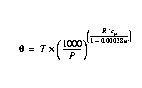(EQ 2)

### Lifting Condensation Level, LCL

The Lifting Condensation Level (LCL) is the level where condensation (saturation) occurs if one lifts an unsaturated surface parcel dry-adiabatically. Graphically on the skew-T plot it is the point where the dry adiabat (originating at the parcel temperature) and mixing ratio lines (originating at the parcel dew point temperature) intersect. In the case of the SUDS analysis the surface parcel temperature and dew point are taken as the average of that data over the lowest 50 mb of a sounding.

The lifting condensation level temperature, from Bolton (1980) is given by: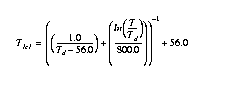EQ 3

where,
· T is the ambient temperature in degrees K.

· Td is the dew point temperature in degrees K.

### Lifted Index, LI

The lifted index is a stability parameter defined as the difference between the 500 mb temperature and the temperature (at 500 mb) of a parcel lifted from the surface dry adiabatically to the condensation level and then moist adiabatically to 500 mb. Positive values reflect stable conditions, negative values reflect unstable conditions.

### Level of Free Convection, LFC

The Level of Free Convection is the level at which a parcel lifted from the surface (defined by a 50 mb average in the SUDS analysis) becomes positively buoyant. The actual temperature at the lifting condensation level (LCL) is typically warmer (to the right on the skew-T plot) than the parcel LCL temperature itself. Thus on the skew-T plot, the temperature trace is to the right of the moist adiabat originating at the LCL temperature and pressure. The point above the LCL at which the temperature trace crosses and stays to the left of that moist adiabat is the Level of Free Convection (LFC).

### Convective Inhibition, CIN

The Convective Inhibition is the amount of energy required to lift a surface parcel (defined by a 50 mb average in the SUDS analysis) to the LFC. Graphically, this energy is proportional to the area on the skew-T plot below the LFC bounded by the environmental temperature profile and the adiabatic lapse rate (dry and wet) of a lifted surface parcel.

### Convective Available Potential Energy, CAPE

The Convective Available Potential Energy (CAPE) is the amount of energy available to a surface parcel that has reached its LFC. Graphically on a skew-T plot, in an unstable situation, above the LFC, the moist adiabat originating at the parcel's LCL temperature will be to the right of the measured temperature trace. At some point a stable layer will be encountered (perhaps not until the tropopause) strong enough such that the temperature trace will cross back to the right of the moist adiabat. The area between the temperature trace and the moist adiabat below the capping stable layer and above the LFC is proportional to the Convective Available Potential Energy (CAPE).

### Vertical Wind Shear, SHEAR

The value calculated is the vertical shear in magnitude of the horizontal wind over the lowest 6 kilometers of the sounding.

### Convective Bulk Richardson Number, R

The convective bulk Richardson number is a relationship between wind shear and buoyancy and is defined as follows: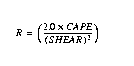(EQ 4)
` `